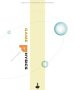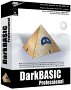# Table Top Physics - Goal

Here is a really good example from Kevin Pegrume. It is an example of a collision with both linear and angular motion, but keeping the maths as simple as possible.

 More table top physics - Hey, you started it :)I hope this little gif helps visualise the tabletop goal example. The view is from above the table. The two rectangles represent two bookends or whatever to make a makeshift "goal". The pencil is initially flat on the smooth table parallel to the goal mouth. The right end marked B is "flicked" towards the right hand post. Lets say the initial velocity of B is 2 m/s. The centre marked CG appears to move at the average initial velocity of A and B.  V(cg)=((2+0)/2) in this case. The rotational velocity from the point of view of appears to be (V(a) - V(b)) depending on the sign convention you are using. Notice that B comes to a stop half way there and is back up to 2 m/s by the time it hits the post. A has now come to a stop and the reaction impulse on B  creates a mirror image of the start conditions and the pencil retraces it tracks. This of course only happens if A and B are parallel to the goal mouth at impact. If the posts are moved a bit closer or further away then one end may be "ahead" of the other at impact. This situation is a bit more complex but I think the calculations can be simplified by considering the "instantaneous" linear velocities of the tips at impact. Work out the resultant reaction impulse and calculate the new rotational and linear velocity from there. Now launch the pen from the same position but this time flick the right end of the pen at the right post. Depending on distance and rotational velocity one extreme end (representing one of our ideal spheres) will contact the goal post first and the pen returns exactly on the same path it launched at as concluded above. Occasionally the centre of the spinning pen enters the goal mouth before one end impacts the corner of the goalpost. The rebound of the pen seems to be approximately perpendicular to the long axis of the pen at impact. In this case it is not one of the ideal spheres that impacts but the rigid connecting rod. It is interesting to note that above a certain threshold angular velocity it is impossible to get the pen into the "back of the net" if the spinning pen clips a goal post. If the angular velocity to rebound angle relationship can be determined (and it does not appear to be too complicated) I am sure this would be a useful formula for approximating collisions calculations in general.

Assume:

velocity of CG = 1 m/s

distance between A and B = 1 m (big pen, I know, but it makes the maths easier)

distance between start point and goal = 2 m

time to travel to goal = 2 s

rotation speed = 1 rotation per 2 seconds = 2*PI/2 radians per second = PI radians per second

# Calculation of impulse at collision:

## First case

assume total mass of 1 Kg concentrated at A and B (i.e. 0.5 Kg at A and 0.5 Kg at B connected by rigid, massless link).

Calculate impulse in two ways, using a numerical method (sum of effect of each mass) and analytically (add impulse caused by linear and angular movement).

### Taking Each Mass Seperately

At collision change of momentum of point A = 0.

At collision change of momentum of point B = from 2 m/s to -2 m/s = 4 m/s * 0.5 Kg = 2 Kg m/s

So total change of momentum = 2 Kg m/s

### In terms of Linear And Rotational Momentum

Linear momentum:

change in liner momentum = change of momentum of CG (1 Kg from 1m/s to -1 m/s) = 2 Kg m/s

Angular momentum:

angular momentum before = mass * radius^2 * angular velocity

= (2*0.5)Kg * (0.5)^2 m²* PI /s

= 0.25* PI Kg m² /s

so change in angular momentum = 0.5* PI Kg m² /s

The impulse required to change this angular momentum is

## Second case

assume total mass of 1 Kg distributed evenly between A and B (i.e. 1 Kg per metre).

Calculate impulse in two ways, using a numerical method (sum of effect of each mass) and analytically (add impulse caused by linear and angular movement).

### Taking Each Mass Seperately

At collision change of momentum = ∫(2*x) dx

where x = distance along pen from point A

So total change of momentum = 2 Kg m/s

### Analytical method

I'll leave this for you to do.

Where I can, I have put links to Amazon for books that are relevant to the subject, click on the appropriate country flag to get more details of the book or to buy it from them.Game Physics - This book has some useful stuff, its more of a textbook, not a step by step guide (although it does have a disc with a lot of C++ code). About the first third of the book is a physics textbook with theoretical exercises, the middle bit covers physics engine topics, and the last third of the book covers mathematical topics. I think I would use this book as a reference book to lookup the theory behind something I might be working on rather than a book to work through in order.

Commercial Software Shop

Where I can, I have put links to Amazon for commercial software, not directly related to the software project, but related to the subject being discussed, click on the appropriate country flag to get more details of the software or to buy it from them.Dark Basic Professional Edition - It is better to get this professional editionThis is a version of basic designed for building games, for example to rotate a cube you might do the following: make object cube 1,100 for x=1 to 360 rotate object 1,x,x,0 next x

This site may have errors. Don't use for critical systems.